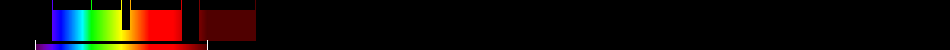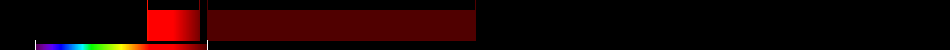# Information for Sensor and Index

## Formula

General Formula $\frac{{\mathrm{NIR}}}{{\mathrm{RED}}+\frac{{b}}{{a}}}$ $\frac{{\mathrm{MS4_Near_Infrared}}}{{\mathrm{MS3_Red}}+\frac{{b}}{{a}}}$ Automatic

## Sensor

Name DubaiSat-1 5 420-890 2.5-5 98.13 Emirates Institution of Advanced Science and Technology 2009-07-29 yes

## Index

Name Soil-adjusted vegetation index 2 SAVI2 $\frac{{\mathrm{NIR}}}{{\mathrm{RED}}+\frac{{b}}{{a}}}$ The actual gain (a) and intercept (b) values of the soil line rather than assuming them to be 1 and 0, respectively 640:760,780:1400 Original formula

## References